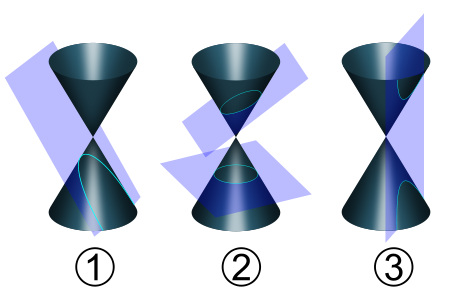# Equations of conic sections

Here we will have a look at three different conic sections:### 1. Parabola

The parabola is a conic section, the intersection of a right circular conical surface and a plane parallel to a generating straight line of that surface. The equation for a parabola is

$y=a(x-b)^{2}+c\; or\; x=a(y-b)^{2}+c$

### 2. Circles and ellipses

The equation of a circle with center at (a,b) and radius r units is

$(x-a)^{2}+(y-b)^{2}=r^{2}$

An ellipse is the figure consisting of all points in the plane whose coordinates satisfy the equation

$\frac{x^{2}}{a^{2}}+\frac{y^{2}}{b^{2}}=1$

If the ellipse has its center at (m,n) the equation could be written as

$\frac{(x-m)^{2}}{a^{2}}+\frac{(y-n)^{2}}{b^{2}}=1$

### 3. Hyperbolas

A hyperbola is a curve, specifically a smooth curve that lies in a plane, which can be defined either by its geometric properties or by the kinds of equations for which it is the solution set. A hyperbola has two pieces, called connected components or branches, which are mirror images of each other and resembling two infinite bows.

The equation of a hyperbola with a center at (m,n) is

$\frac{(x-m)^{2}}{a^{2}}-\frac{(y-n)^{2}}{b^{2}}=1$

## Video lesson

Write the given equation on the standard equation form for ellipses